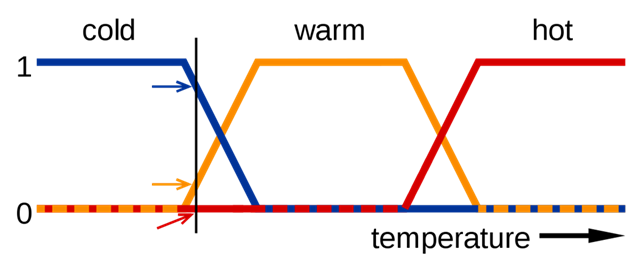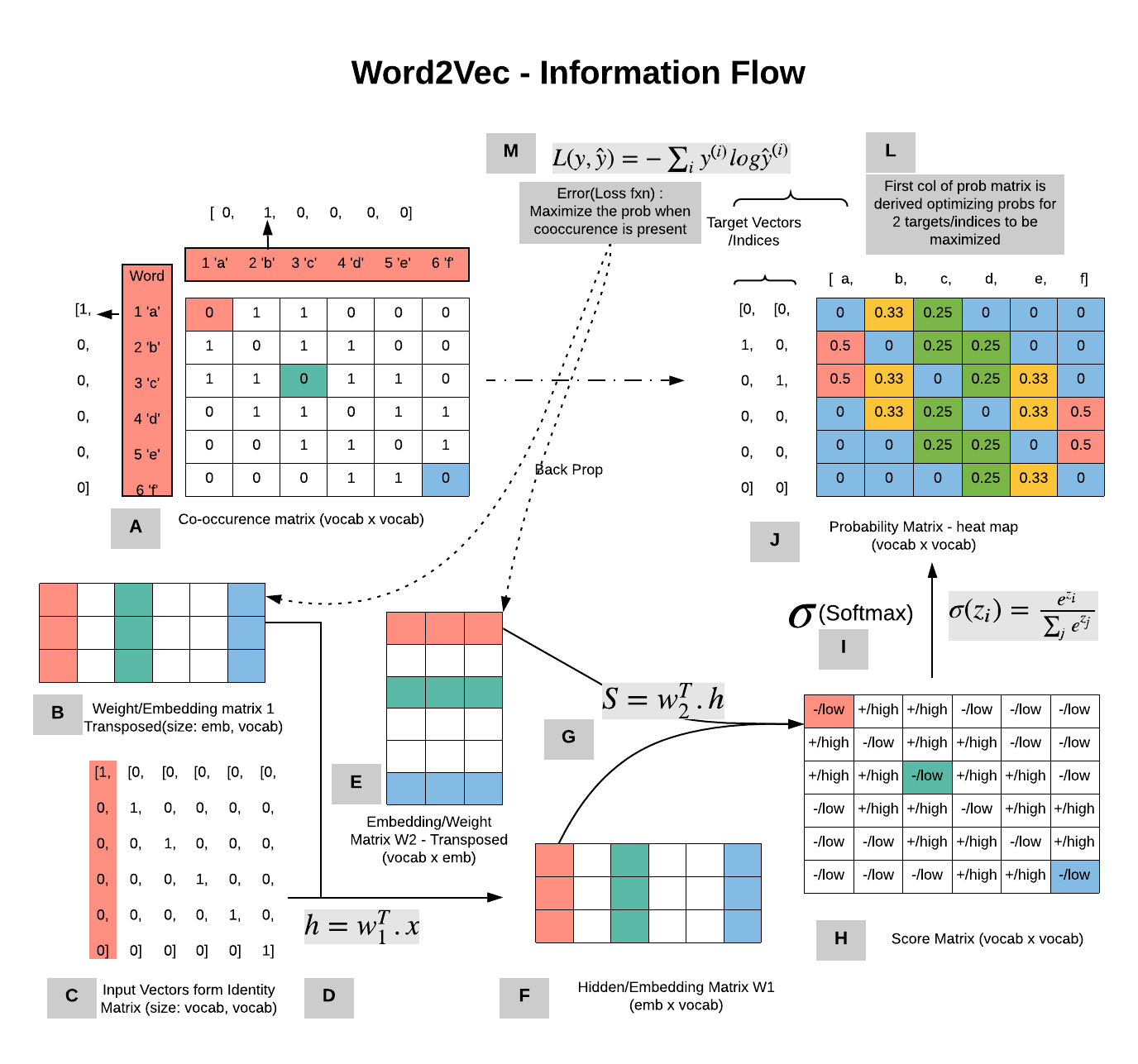## 词向量基础

### One-Hot 编码（独热编码）

'中国'表示为：[00010000000.....]
'美国'表示为：[0000000010000...]

• 容易受维数灾难的困扰，尤其是将其用于 Deep Learning 的一些算法时：词汇表一般都非常大，比如达到百万级别，这样每个词都用百万维的向量来表示简直是内存的灾难。这样的向量其实除了一个位置是1，其余的位置全部都是0，表达的效率不高
• 不能很好地刻画词与词之间的相似性：任意两个词之间都是孤立的，这样使得算法对相关词的泛化能力不强。因为任何两个one-hot向量之间的内积都是0，很难区分他们之间的差别。光从这两个向量中看不出两个词是否有关系，哪怕是丈夫和老公这样的同义词也不能幸免于难。

### 分布式词向量/词嵌入

Distributed representation可以解决One hot representation的问题，它最早是 Hinton 于 1986 年提出的。它的思路是通过训练，将每个词都映射到一个较短的词向量上来。所有的这些词向量就构成了向量空间，进而可以用普通的统计学的方法来研究词与词之间的关系。这就是word embedding（词嵌入），即指的是将词转化成一种分布式表示，又称词向量。分布式表示将词表示成一个定长的连续的稠密向量，这个较短的词向量维度大小一般需要在训练时自己来指定。

Distributed Representation 可以创建多个层次结构或分段，其中可以为每个单词显示的信息分配不同的权重。这些分段或维度的选择可以是我们决定的，并且每个单词将由这些段中的权重分布表示。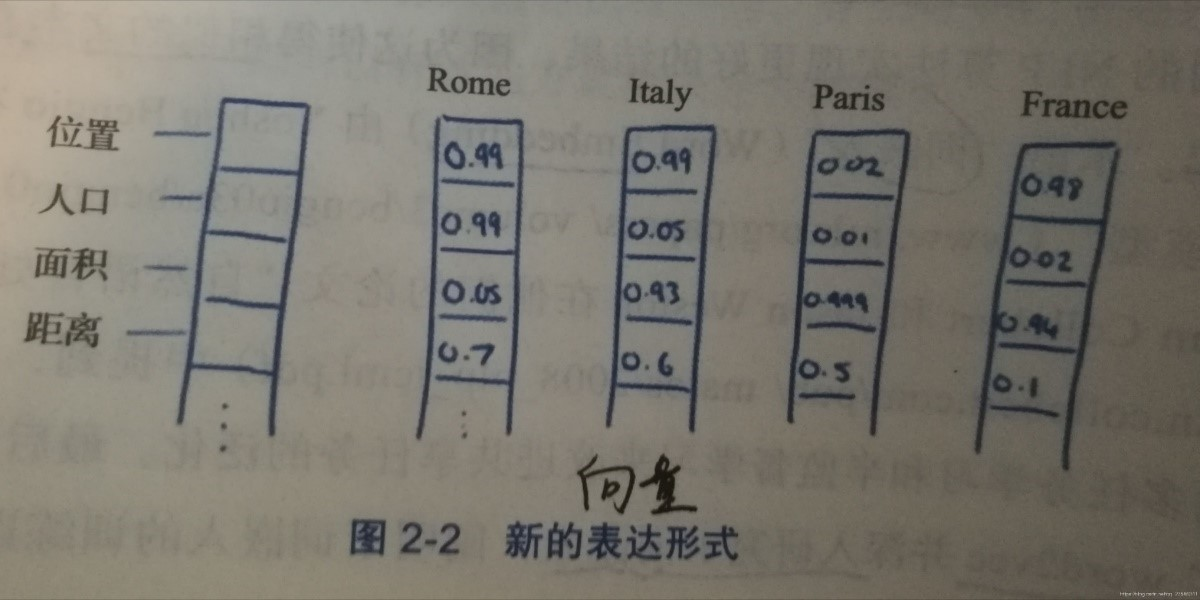• 词之间存在相似关系：是词之间存在“距离”概念，这对很多自然语言处理的任务非常有帮助。
• 能够包含更多信息，并且每一维都有特定的含义。在采用one-hot特征时，可以对特征向量进行删减，词向量则不能。

### 词向量可视化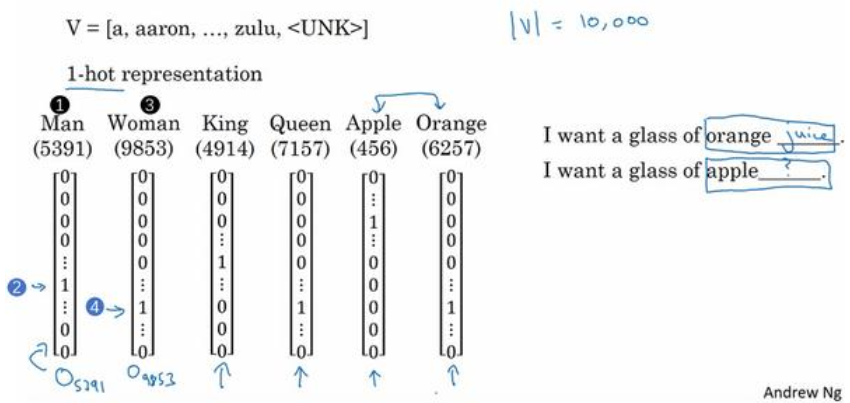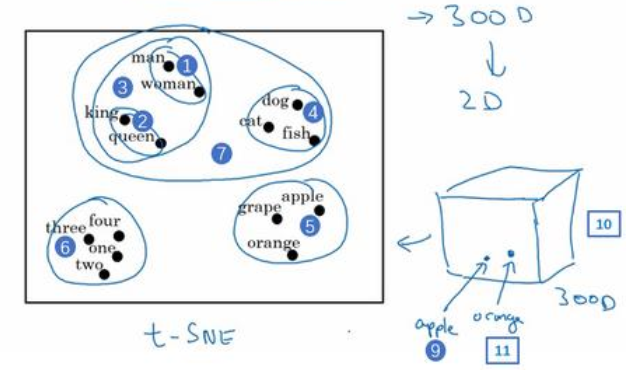### 词向量的作用

• 先从大量的文本集中学习词向量，或者可以下载网上预训练好的词向量模型。
• 把这些词向量模型迁移到新的只有少量标注训练集的任务中。
• 考虑是否微调，用新的数据调整词向量。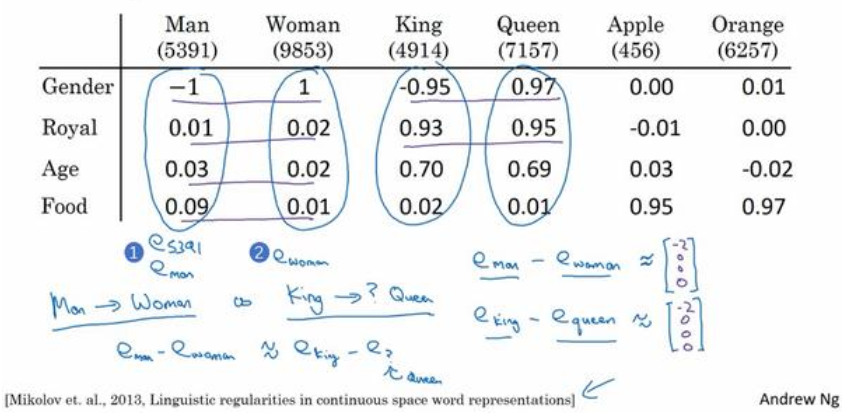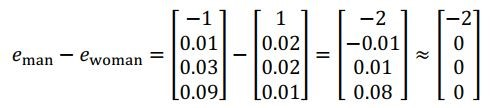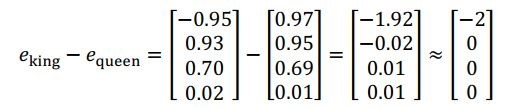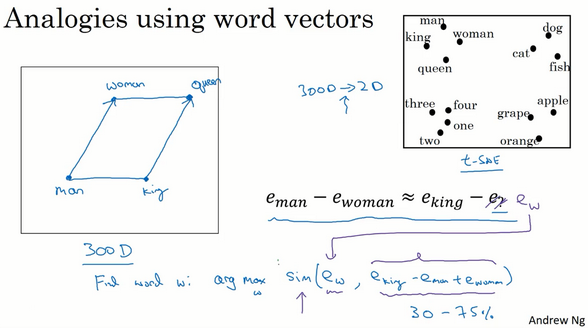$$\text {Find word w: argmax } \mathrm{sim}(e_{w}, e_{king}-e_{man}+e_{woman})$$

## 词向量生成方式

### 基于统计方法

• I like deep learning.
• I like NLP.
• I enjoy flying.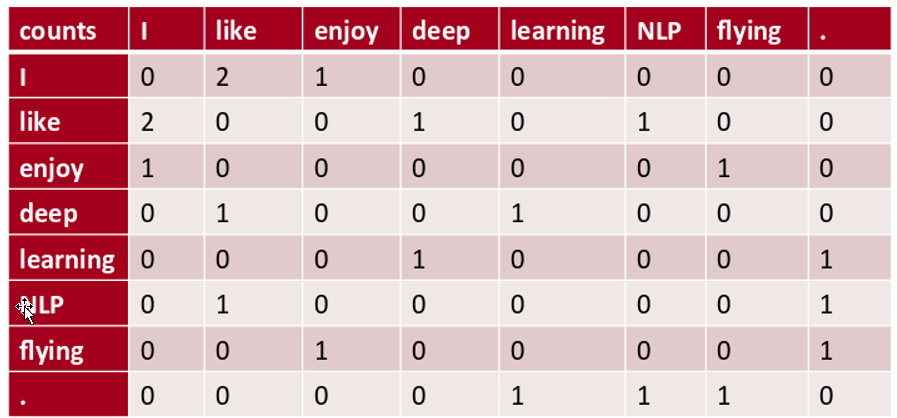SVD（奇异值分解）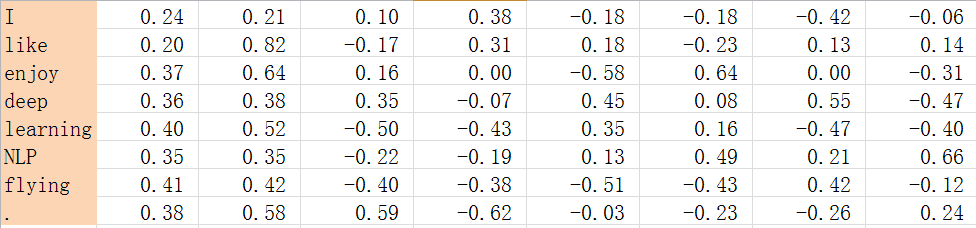SVD得到了word的稠密（dense）矩阵，该矩阵具有很多良好的性质：语义相近的词在向量空间相近，甚至可以一定程度反映word间的线性关系。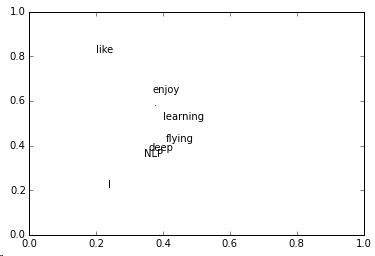$$P(sen=(w_1, w_2, .., w_m)) = P(w_1)P(w_2|w_1)P(w_3|w_2,w_1)…P(w_m|w_{m-1}..w_1)$$

$$P(w_t|w_1,w_2,..,w_{t-1})=P(w_t|w_{t-1}, w_{t-2}…w_{t-(n+1)})$$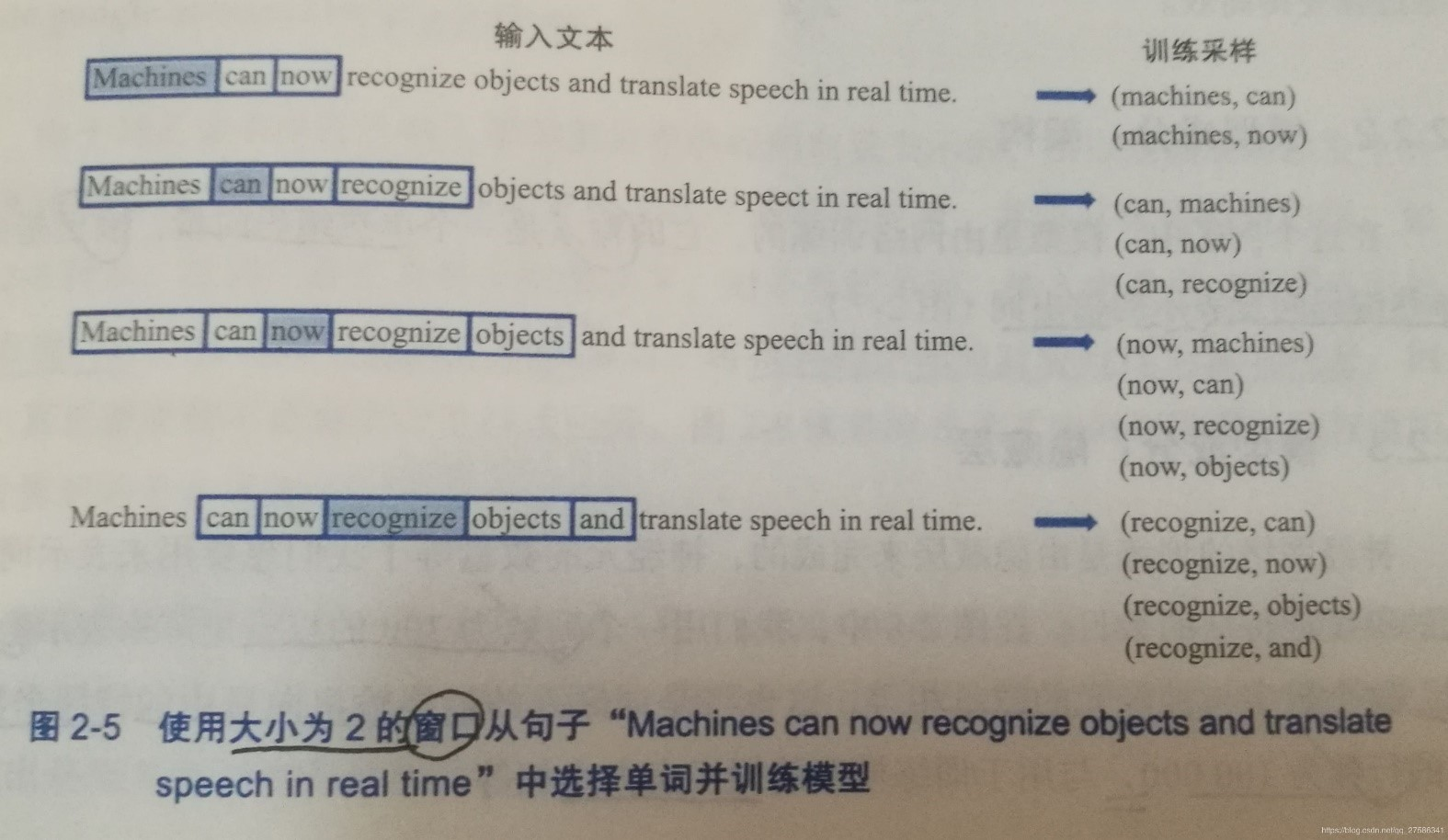Bengio通过下面的一个三层神经网络来计算：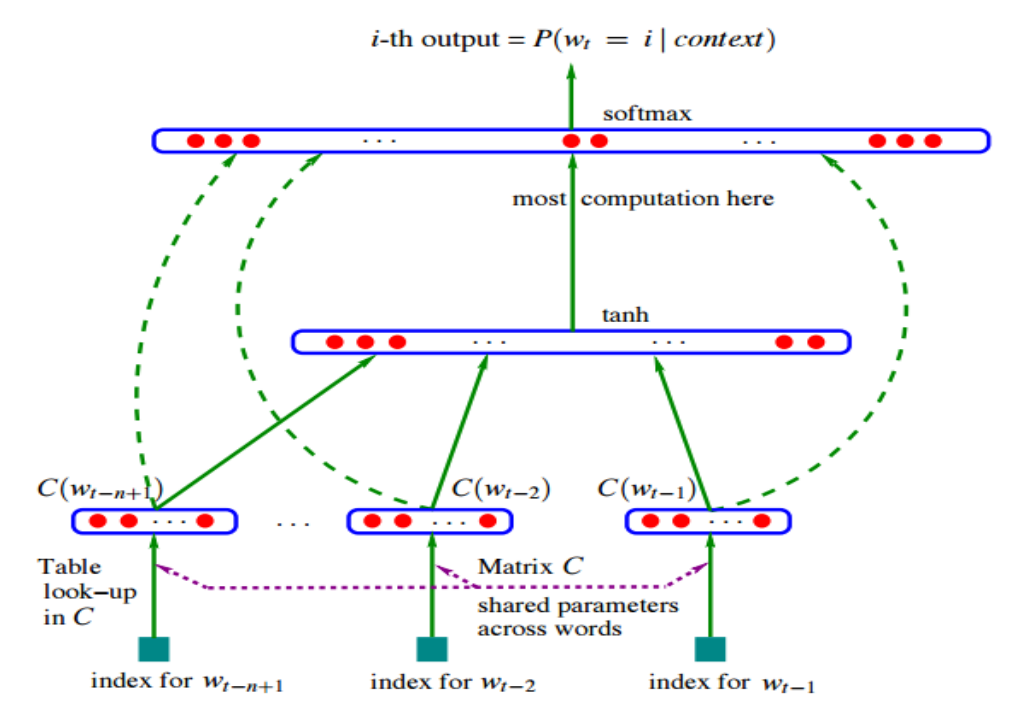## CBOW与Skip-Gram

### 用于神经网络语言模型的CBOW与Skip-Gram

CBOW模型，利用上下文或周围的单词来预测中心词。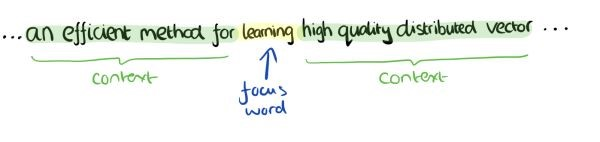CBOW（Continuous Bag-of-Words Model）：在已知上下文，例如$w_{t-2},w_{t-1},w_{t+1},w_{t+2}$的前提下，如何预测当前词$w_t$。学习的目标函数是最大化对数似然函数：

$$\sum_{w\in C}logp(w|Context(w))$$

Skip-gram模型，使用中心词来预测上下文词。

Skip-Gram模型和CBOW的思路是反着来的（互为镜像），即输入是特定的一个词的词向量（单词的one-hot编码），而输出是特定词对应的上下文词向量（所有上下文单词的one-hot编码）。还是上面的例子，我们的上下文大小取值为4， 特定的这个词”Learning”是我们的输入，而这8个上下文词是我们的输出。

Skip-gram（Continuous Skip-gram Model）：在已知当前词$w_t$的前提下，预测其上下文，例如$w_{t-2},w_{t-1},w_{t+1},w_{t+2}$。目标函数形如：

$$\sum_{w\in C}logp(Context(w)|w)$$

CBOW和Skip-gram均为三层神经网络（输入层、投影层和输出层），而上下文的词的具体个数是可定义的。窗口大小为5时，两个模型的网络结构如下：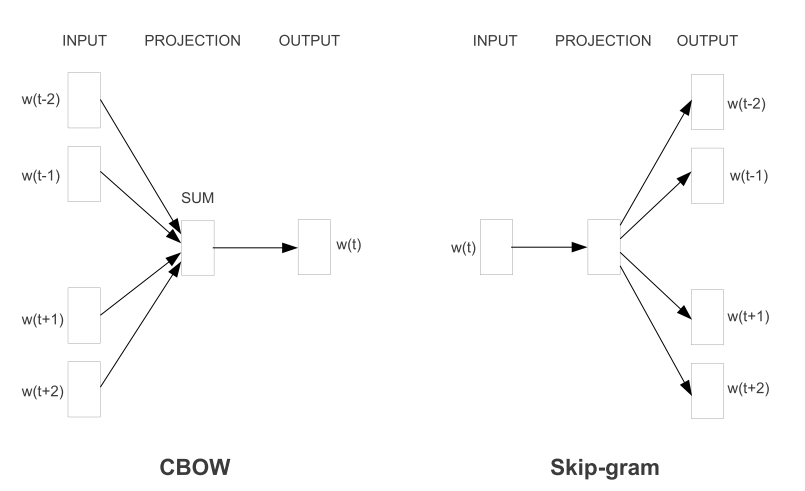### Huffman树

Huffman树是一种特殊结构的二叉树，由Huffman树设计的二进制前缀编码，也称为Huffman编码，在通信领域有着广泛的应用。在word2vec模型中，在构建层次Softmax的过程中，也使用到了Huffman树的知识。

Huffman树的基本概念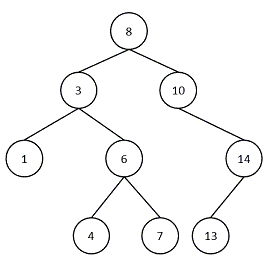• 路径：在一棵树中，从一个节点到另一个节点之间的分支构成的通路，如从节点8到节点1的路径如图所示：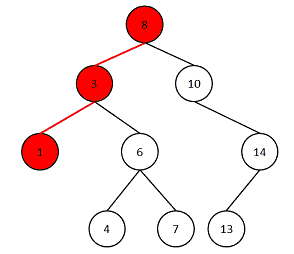• 路径长度：路径上分支的数目，在上图中，路径长度为2。
• 节点的权：为树中的每一个节点赋予的一个非负的值，如上图中每一个节点中的值。
• 节点的带权路径长度：从根节点到该节点之间的路径长度与该节点权的乘积：如对于1节点的带权路径长度为：2。
• 树的带权路径长度：所有叶子节点的带权路径长度之和。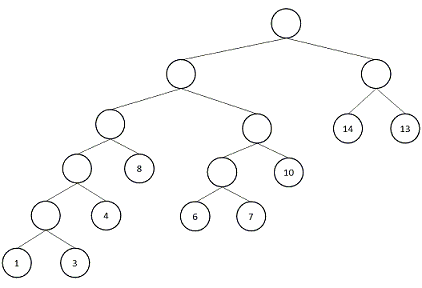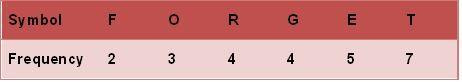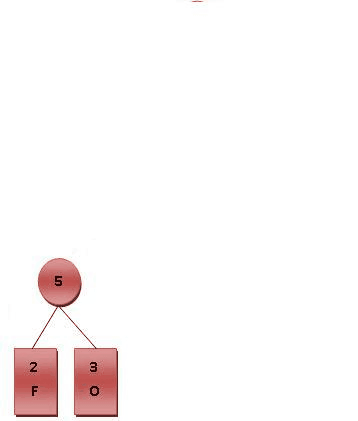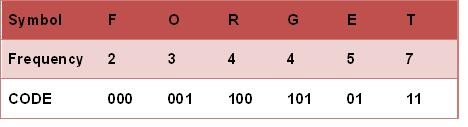• 进行霍夫曼编码前，我们先创建一个霍夫曼树
• 将每个英文字母依照出现频率由小排到大，最小在左
• 每个字母都代表一个终端节点（叶节点），比较O.R.G.E.T六个字母中每个字母的出现频率，将最小的两个字母频率相加合成一个新的节点。如Fig.2所示，发现F与O的频率最小，故相加2+3=5
• 比较R.G.E.T，发现R与G的频率最小，故相加4+4=8
• 比较8.E.T，发现5与E的频率最小，故相加5+5=10
• 比较10.T，发现8与T的频率最小，故相加8+7=15
• 最后剩15，没有可以比较的对象，相加10+15=25
• 最后产生的树状图就是霍夫曼树
• 进行编码
• 给霍夫曼树的所有左链接’0’与右链接’1′
• 从树根至树叶依序记录所有字母的编码

### word2vec中的CBOW与Skip-Gram

word2vec也使用了CBOW与Skip-Gram来训练模型与得到词向量，但是并没有使用传统的DNN模型，而是对其进行了改进。最先优化使用的数据结构是用霍夫曼树来代替隐藏层和输出层的神经元。

• 叶子节点：起到输出层神经元的作用，叶子节点的个数即为词汇表的大小。
• 内部节点：起到隐藏层神经元的作用。

CBOW模型

• 第一层是输入层, 输入已知上下文的词向量；
• 中间层称为线性隐含层, 它将所有输入的词向量累加；
• 第三层是一棵哈夫曼树, 树的的叶节点与语料库中的单词一一对应, 而树的每个非叶节点是一个二分类器(一般是softmax感知机等), 树的每个非叶节点都直接与隐含层相连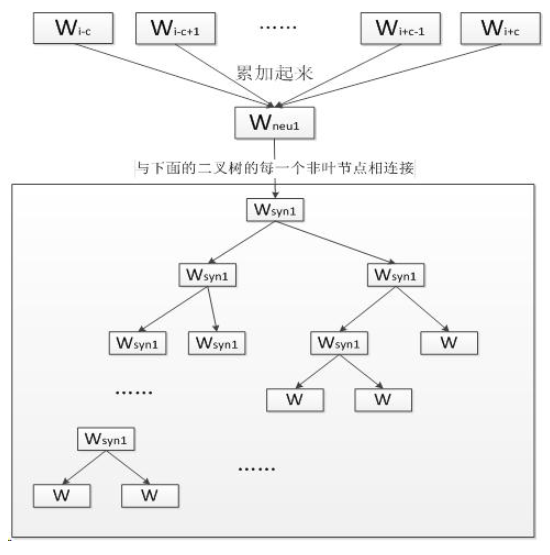• 根据预料库建立词汇表, 词汇表中所有单词拥有一个随机的词向量。我们从语料库选择一段文本进行训练
• 将单词W的上下文的词向量输入CBOW, 由隐含层累加, 在第三层的哈夫曼树中沿着某个特定的路径到达某个叶节点, 从给出对单词W的预测
• 根据W的哈夫曼编码确定从根节点到叶节点的正确路径, 也确定了路径上所有分类器应该作出的预测
• 采用梯度下降法调整输入的词向量, 使得实际路径向正确路径靠拢。在训练结束后从词汇表中得到每个单词对应的词向量

Skip-gram模型

Skip-gram模型同样是一个三层神经网络，skip-gram模型的结构与CBOW模型正好相反，skip-gram模型输入某个单词，输出对它上下文词向量的预测。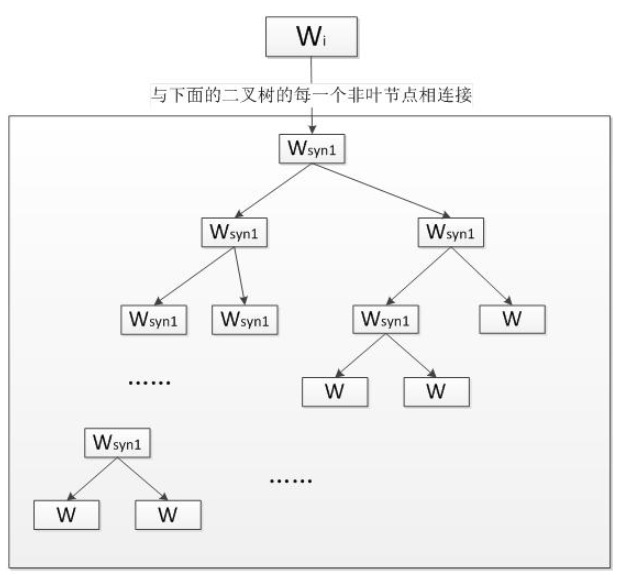Skip-gram的核心同样是一个哈夫曼树, 每一个单词从树根开始到达叶节点，可以预测出它上下文中的一个单词。对每个单词进行N-1次迭代，得到对它上下文中所有单词的预测, 根据训练数据调整词向量得到足够精确的结果。

## Word2vec对神经语言模型的改进

### Hierarchical Softmax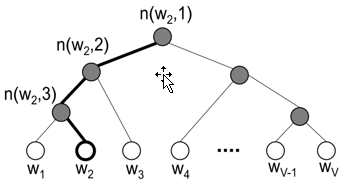$$P(n, left ) = \sigma({v^{‘}_{w}} \cdot \boldsymbol{h})$$

$$P(n, right) =1-\sigma(v^{‘}_w \cdot \boldsymbol{h}) = \sigma(-v_{w}^{‘} \cdot h)$$

$$P(w=w_O|w_I)= \Pi_{j=1}^{L(w)-1}P(\sigma(I(n(w,j+1==left) v^{‘}_{w} \cdot \mathbf{h}))$$

$$P(w_2=w_O) = P(n(w_2,1), left) \cdot P(n(w_2,2), left) \cdot P(n(w_2, 3), right) = \sigma({v^{‘}_{n(w_2,1)}}^T \mathbf{h}) \cdot \sigma({v^{‘}_{n(w_2,2)}}^T \mathbf{h}) \cdot \sigma(-{v^{‘}_{n(w_2,3)}}^T \mathbf{h})$$

$$\sum\limits_{j=1}^{V}P(w_j=w_O)=1$$

$$E = -\log P(w=w_O|w_I) = – \sum\limits_{j=1}^{L(w)-1}\log \sigma([I] {v^{‘}_{j}}^{T} \mathbf{h})$$

$$\frac{\partial E}{\partial v^{‘}_j \mathbf{h}} = (\sigma([I] {v_{j}^{‘}}^T \mathbf{h}))[I]$$

$$\frac{\partial E}{\partial v^{‘}_j} = \frac{\partial E}{\partial {v^{‘}_{j}}^T \mathbf{h}} \frac{\partial {v^{‘}_{j}}^T \mathbf{h}}{\partial {v^{‘}_{j}}^T} = (\sigma( {v^{‘}_{j}}^T \mathbf{h}) – t_j) \mathbf{h}$$

$${v^{‘}_{j}}^{(new)} = {v^{‘}_{j}}^{(old)} – \eta (\sigma( {v^{‘}_{j}}^T \mathbf{h}) – t_j) \mathbf{h},\ j=1,2,3,…,L(w)-1$$

$$\frac{\partial E}{\partial \mathbf{h}} = \sum\limits_{j=1}^{L(w)-1} \frac{\partial E}{\partial {v^{‘}_{j}}^T \mathbf{h}} \frac{\partial {v^{‘}_{j}}^T \mathbf{h}}{\partial \mathbf{h}} =\sum\limits_{j=1}^{L(w)-1}(\sigma( {v^{‘}_{j}}^T \mathbf{h}) – t_j)\cdot v^{‘}_{j}$$

### Negative Sampling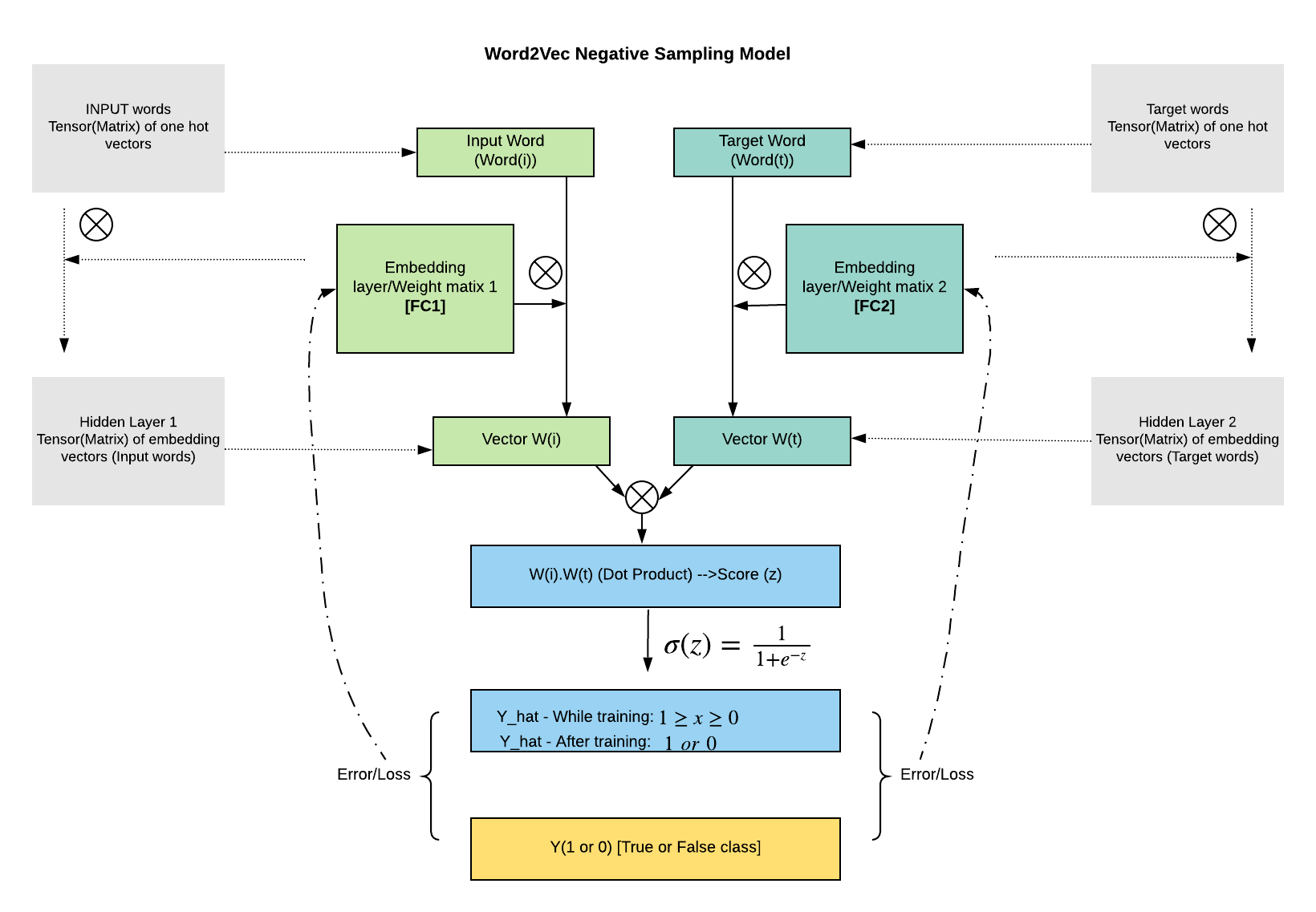$$weight(w) = \frac{coun(w)^{0.75}}{\sum _{u}count(w)^{0.75}}$$

$$E = -\log \sigma(v^{‘}_{w_O}\mathbf{h}) – \sum\limits_{w_j \in \mathcal{W}_{neg}}\log \sigma(-v^{‘}_{w_j}\mathbf{h})$$

$\mathcal{W}_{neg}$是负例单词集合。上述公式跟原本的负采样的有些许差别，具体的推导细节可以参考这篇Goldberg, Y. and Levy, O. (2014). word2vec explained: deriving mikolov et al.’s negative- sampling word-embedding method. 这里不再赘述了。 只需要记住，NS也是对softmax函数做的优化，除了word2vec，在其他地方涉及到softmax的均可以采用类似的思想来重写目标函数。

$$\frac{\partial E}{\partial {v^{‘}_{j}}^T \mathbf{h}} = \sigma( {v^{‘}_{j}}^T \mathbf{h}) – t_j$$

$${v^{‘}_{j}}^{(new)} = {v^{‘}_{j}}^{(old)} – \eta (\sigma( {v^{‘}_{j}}^T \mathbf{h}) – t_j) \mathbf{h},\ w_j \in \{w_O \} + \mathcal{W}_{neg}$$

• HS实现比较复杂， 需要借助哈夫曼树，而且HS是专门优化softmax的方法
• NS相对简单，仅仅是简单采样，NS则是一种概率采样的方法，属于NCE(Noise Contrastive Estimation)的一种简单形式，代码实现也很简单。训练得到的词向量质量也很高，相对常用一些。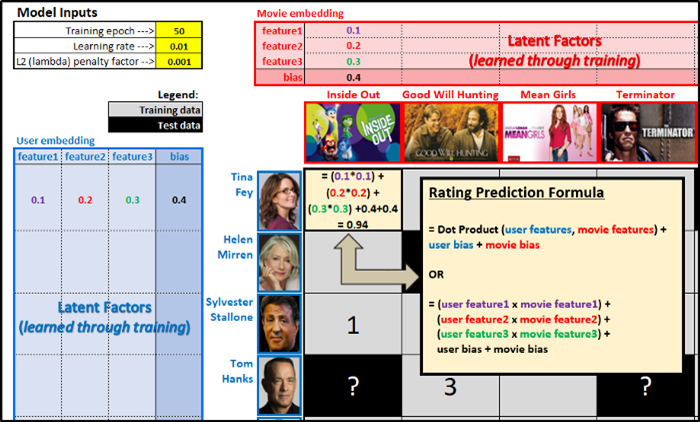##### 使用Excel搭建推荐系统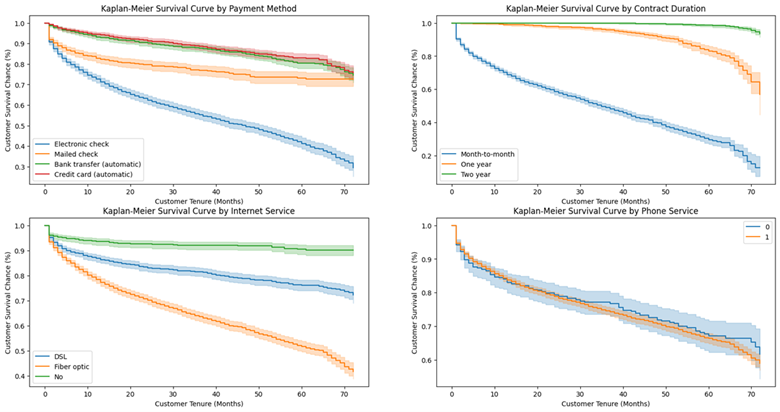##### 生存分析从概念到实战